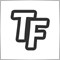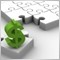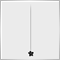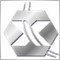# How to avoid zero divide before indicator calculates17183

Hello,

I have an indicator I'm working on that when it is first placed on the chart, the bollinger band values are zero and I end up with a zero divide message in both MT4 and MT5.  After the bollinger band values and the indicator have a chance to calculate, it displays correctly but appears again when i change the chart or timeframe (and sometimes the indicator doesn't display after the change).

What can I do to avoid the zero divide error?  Is there a wait until loaded function?  Or should I put the division calculation in an if statement to wait until the bollinger values are above zero?

Thank you10371

Ahhh, the old MetaTrader divide by zero issue (https://www.mql5.com/en/search#!keyword=zero%20divide&module=mql5_module_forum&method=2

There's no "wait until loaded" function that I know of. But the if statement you suggested should fit the bill. Or maybe use IndicatorCounted function?45347

Don't know is this applicable in your case, but universal killer for "zero divide" is increasing of divider by insufficient value. Example:

```if(Group3_Bar_3_Body == SIZE_LONGER) {
if(fabs(Open[i_Pivot_Bar] - Close[i_Pivot_Bar]) / (0.000000001 + High[i_Pivot_Bar] - Low[i_Pivot_Bar]) <= 0.5) continue;
} else if(Group3_Bar_3_Body == SIZE_SHORTER) {
if(fabs(Open[i_Pivot_Bar] - Close[i_Pivot_Bar]) / (0.000000001 + High[i_Pivot_Bar] - Low[i_Pivot_Bar]) >= 0.5) continue;
}
```17183

Thanks for the replies.  To close this out for anyone that might search this zero divide situation in the future, it wasn't because of the data not loading before the indicator calculated.  It was because the bollinger bands really were zero value for the 20 bars before they started to calculate.  I added an if statement and that solved the issue.  No more zero divide.

This screenshot shows the beginning of the chart where the bollinger bands do not calculate until the Moving Average has all the bars it needs.25423

tfworks.com:

Hello,

I have an indicator I'm working on that when it is first placed on the chart, the bollinger band values are zero and I end up with a zero divide message in both MT4 and MT5.  After the bollinger band values and the indicator have a chance to calculate, it displays correctly but appears again when i change the chart or timeframe (and sometimes the indicator doesn't display after the change).

What can I do to avoid the zero divide error?  Is there a wait until loaded function?  Or should I put the division calculation in an if statement to wait until the bollinger values are above zero?

Thank you

Simply check if you divide on zero before divide.

for example:

A = B / C

change it to:

if(C>0) A = B / C1420

tfworks.com:

Hello,

I have an indicator I'm working on that when it is first placed on the chart, the bollinger band values are zero and I end up with a zero divide message in both MT4 and MT5.  After the bollinger band values and the indicator have a chance to calculate, it displays correctly but appears again when i change the chart or timeframe (and sometimes the indicator doesn't display after the change).

What can I do to avoid the zero divide error?  Is there a wait until loaded function?  Or should I put the division calculation in an if statement to wait until the bollinger values are above zero?

Thank you

the official RSI.mq4 do like:

```      if(ExtNegBuffer[InpRSIPeriod]!=0.0)
ExtRSIBuffer[InpRSIPeriod]=100.0-(100.0/(1.0+ExtPosBuffer[InpRSIPeriod]/ExtNegBuffer[InpRSIPeriod]));
else
{
if(ExtPosBuffer[InpRSIPeriod]!=0.0)
ExtRSIBuffer[InpRSIPeriod]=100.0;
else
ExtRSIBuffer[InpRSIPeriod]=50.0;
}```999

Also discussed here

https://www.mql5.com/en/forum/32245zero divide
• www.mql5.com
I have a question about zero divide in indicator when it run. - - Category: technical indicators6

Ahmed Soliman:

Simply check if you divide on zero before divide.

for example:

A = B / C

change it to:

if(C>0) A = B / C

You are My genius,  You solved my Bunch of problems, I can't Stop Crying.